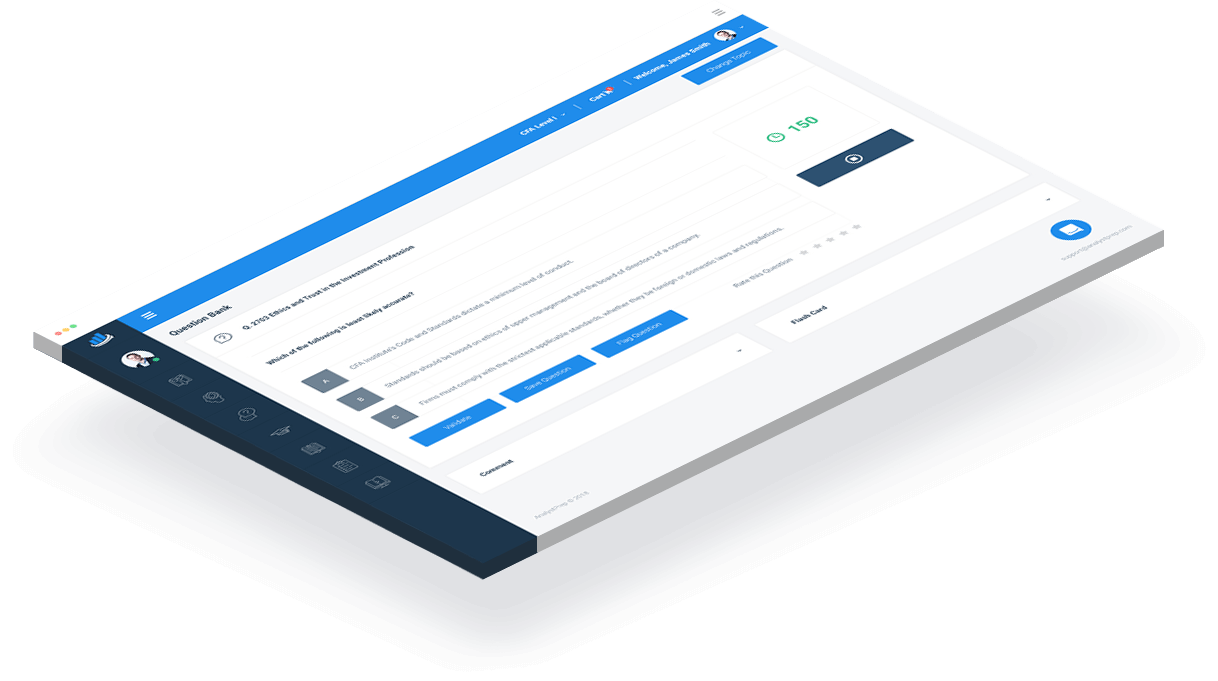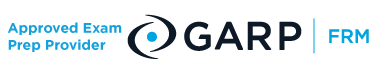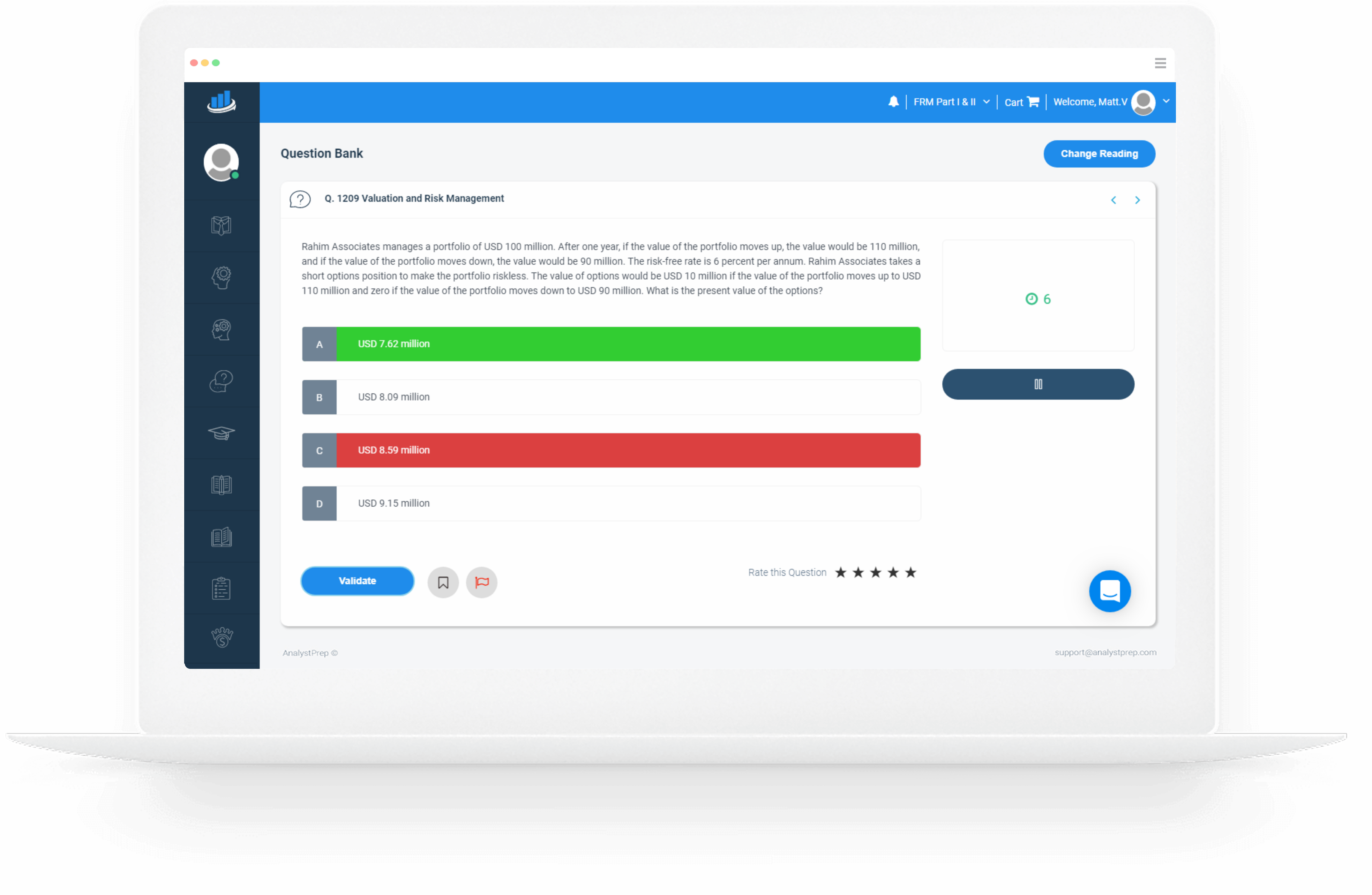### FRM Part II Practice Questions

#### Only Hard, High-Quality Questions# There are no Shortcuts – Practice is Key

Our FRM Part II practice questions are designed to strengthen your understanding of key concepts and gauge your preparation with respect to your peers around the world.

Each question in the FRM Part II QBank has been developed by certified FRMs to ensure the highest level of quality and relevance. As a sign of trust, you get exclusive access to hundreds of FRM Part II practice questions as soon as you sign up. Thousands of candidates have used the question bank, with over 1 million questions answered.

Our practice questions are updated every year to ensure consistency with the most recent curriculum as outlined by the GARP.

AnalystPrep’s game-changing algorithms enable you to compare your scores with those of other candidates around the globe.### Why AnalystPrep?

Our FRM part II practice questions are known, not just for their level of detail but also for their high level of complexity. Preparing using too easy questions is a sure way to get frustrated on the big day, and as much is shared by candidates on feedback forums. At AnalystPrep, we pride ourselves in thorough, continuously challenging questions that only make you a better-prepared candidate.

Our practice questions are subdivided according to readings to help you focus on a particular area at a time. If you need to gauge your knowledge on multiple readings, you can easily create a customized quiz with any number of questions.

We also include a comments section in each question through which you can link up with our experienced instructor for extra tips and tricks.

# Free FRM Part 2 Practice Questions

## Estimating Market Risk Measures

Assuming that the P/L over a specified period is normally distributed and has a mean of 14.1 and a standard deviation of 28.2. What is the 95% VaR and the corresponding 99% VaR?

A) The 95% VaR is 32.289 and the 99% VaR is 51.4932

B) The 95% VaR is 36.495 and the 99% VaR is 51.556

C) The 95% VaR is 55.236 and the 99% VaR is 36.49551

D) The 95% VaR is 36.225 and the 99% VaR is 41.586

Recall that: αVaR = -µP/L + σP/Lzα ,

Therefore, the 95% VaR is: -14.1 + 28.2Z0.95 = -14.1 + 28.2 × 1.645 = 32.289

The 99% VaR is: -14.1 + 28.2Z0.99 = -14.1 + 28.2 × 2.326 = 51.4932

## The Art of Term Structure Models: Volatility and Distribution

James Greenberg, an analyst at HSBC, is employing the Cox-Ingersoll-Ross (CIR) model for the short-term rate process.

His assumptions include:

The time-step is monthly, dt = 1/12, today’s initial rate, r(0) = 2.11%, the annual basis point volatility, sigma = 3.17%, the long-run rate, theta = 7.64%, the strength of reversion, k = 0.57.

For the first month, dw = 0.160. What is the short-rate in the first month under this CIR process, r(1/12)?

A) -3.006%

B) -1.336%

C) 2.446%

D) 3.006%

Recall that from the CIR model, we have:
dr = k (θr)dt + σrdw

From the data provided in the question:
σ = 0.0317, r = 0.0211, θ = 0.0764, k = 0.57, dw = 0.16

Therefore:
dr = 0.57(0.0764 − 0.0211) × 112 + 0.0317√0.0211 × 0.16

dr = 0.00336 = 0.336%

The short rate in the first month under this CIR model is:
2.11% + 0.336% = 2.446%

## Volatility Smiles

Suppose that a small-cap stock is priced at $0.6560. Suppose further that the European call and put options computed by Black-Scholes-Merton model are$0.0249 and $0.0501 respectively. Compute the market price of a FEB$0.75 call option if the market price of a FEB $0.75 put option is$0.0317.

A) $0.0065 B)$0.0025

C) $0.0337 D)$0.0654

For Black-Scholes-Merton model and in the absence of arbitrage opportunities, the put-call parity satisfies:
pBS + S0 e−qT = cBS Ke−rt

For the market prices, put-call parity holds when arbitrage opportunities are absent such that:
pMKT + S0 e−qT = cMKT Ke−rt

The difference between the two equations is:
pBS − pMKT = cBS − cMKT

From the question we have that:
cBS = 0.0249, pBS = 0.051 and pMKT = 0.0317

Thus:
0.0501 − 0.0317 = 0.0249 − cMKT
⇒ cMKT = 0.0065

## Portfolio Credit Risk

A $1 million portfolio of credits is divided into 100 credits with each credit having default probability represented by π. The default correlation is zero, and each credit is equally weighed. If π = 0.03 and the 95th percentile of the number of defaults is given as 4, calculate the Credit VaR. A)$0.01 million

B) $0.004 million C)$0.09 million

D) $0.001 million The correct answer is: A). A default correlation equal to 0 implies the portfolio is a binomial-distributed random variable because there is no correlation with other firms/credits. In this case, the number of defaults would be binomially distributed with n = 100 and θ = 0.03 What’s more each credit has a volume of$10,000 (=$1000,000/100) The expected loss = 1,000,000 × 0.03 = 30,000 If there are 4 defaults, the credit loss is$10,000 × 4 = $40,000 Credit VaR = credit loss – expected loss = 40,000 – 30,000 =$10,000

## Credit Risk Measurement and Management

Raul Gaucho Trading is trading firm from Brazil that needs to have a very quick idea on a swap’s Bilateral Credit Value Adjustment (BCVA).The firm discovered that the expected positive exposure (EPE) for a trade of this type is 13.6% with an expected negative exposure of 0.09. The counterparty credit spread is found out to be around 267 bps and the credit spread of the trader’s own institution is 191 basis points per annum. Which of the following is nearest to an estimate of the BCVA?

A) -32.268 bps

B) -69.326 bps

C) -21.338 bps

D) 19.122 bps

Recall that BCVA, which is an obvious extension of DVA, is calculated by using the following formula:

From the question, we have: EPE = 0.136, Spreadc = 267, ENE = 0.09, and Spreadp = 191

Therefore:

BCVA = 0.136 × 267 bps − 0.09 × 191 bps

= 19.122 bps## Question 2964

Supposing we are given the following information for a loan:

• $89.5 million is the expected revenue •$8.89 million is the operating cost
• $50.98 million is the tax expense •$10 million is the expected loss
• $24.01 million is the return on risk capital •$69.5 million is the economic capital

What is the RAROC for the loan?

A) 0.7867

B) 0.4537

C) 0.6279

D) 0.8794

Recall that:

RAROC = (Expected revenues − Costs − Expected losses − Taxes + Return on risk capital +/− Transfers)/Economic capital

Therefore:

RAROC = (89.5 − 8.89 − 50.98 − 10 + 24.01)/69.5

= 0.6279

## Monitoring Liquidity

A liquidity division in XYZ bank operates a buy/sell back operation. Assume that after 3 months (0.25 years), the bank buys a bond worth 200,000 with an interest rate of 10% and sells it back after 6 months at the forward price. Assume that the purchase price is 99.50. Calculate the amount the bank pays at the start of the contract.

A) 204,000

B) 309,400

C) 403,600

D) 508,300

The amount the bank pays is given by

200,000 × (99.50% + 10% × 0.25) = 204,000

## Alpha (and the Low-Risk Anomaly)

You have been given the following information for a portfolio:

 Portfolio return 10% Beta 1.2 Standard deviation of the portfolio 5% Benchmark return 8% Tracking error 10% Risk-free rate 3%

Calculate the Sharpe and the information ratios for the portfolio.

A) Sharpe ratio: 1.4; Information ratio: 0.2

B) Sharpe ratio: 1.4; Information ratio: 0.7

C) Sharpe ratio: 0.4; Information ratio: 0.2

D) Sharpe ratio: 0.4; Information ratio: 0.7

Sharpe Ratio = (RpRf )/σ

Sharpe Ratio = (10 – 3)/ 5

Sharpe Ratio = 1.4

Information Ratio = (RpRb)/(Tracking error)

Information Ratio = (10 – 8)/10

Information Ratio = 0.2

## Risk Monitoring and Performance Measurement

A fund manager is concerned about his portfolio’s liquidity profile. He does not wish to exceed more than 15 percent of the daily volume in any given security holding. The daily volume of the security is 100,000 while the number of shares held by the manager is 10,000. Which of the following is closest to the liquidity duration of the security?

A) 0.67

B) 0.015

C) 0.15

D) 0.1

Liquidity duration = Qi/(0.15 × Vi)

Qi = Number of shares held in security i
Vi = Daily volume of security i

Liquidity duration = 10,000/(0.15 × 100,000) = 0.67

### FRM®Part 2 Packages

Our FRM® study packages start as low as $249 with a one exam Practice package. Our Learn + Practice packages include study notes and video lessons for$399.

###### / 12-month access
• Question Bank
• Printable Mock Exams
• Performance Tracking Tools